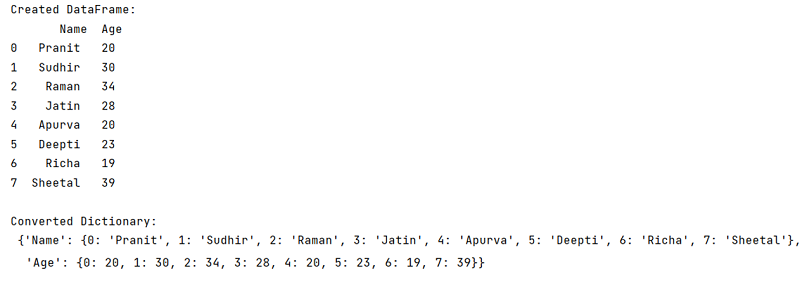# How to Convert a DataFrame to a Dictionary?

Given a Pandas DataFrame, we have to convert it into a Dictionary. By Pranit Sharma Last updated : September 22, 2023

Pandas is a special tool that allows us to perform complex manipulations of data effectively and efficiently. Inside pandas, we mostly deal with a dataset in the form of DataFrame. DataFrames are 2-dimensional data structures in pandas. DataFrames consist of rows, columns, and the data.

Python Dictionary is a collection of data values that is unordered and the data values hold the key:value relationship. The key in the dictionary is unique and holds a single value (homogeneous in nature). Dictionaries in Python are fast and optimized as compared to other data types of Python.

## Problem statement

Given a Pandas DataFrame, we have to convert it into a Dictionary.

## How to Convert a DataFrame to a Dictionary?

To convert a pandas DataFrame into a dictionary, we will use pandas.DataFrame.to_dict() method. It is the most common used method of the DataFrame. The to_dict() method is called with this DataFrame (i.e., with the dataframe's object) and returns the dictionary.

### Syntax

```DataFrame.to_dict(
orient='dict',
into=<class 'dict'>
)
```
Note

To work with pandas, we need to import pandas package first, below is the syntax:

```import pandas as pd
```

Let us understand with the help of an example,

## Python program to convert a DataFrame to a dictionary

```# Importing pandas package
import pandas as pd

# Create d DataFrame
df = pd.DataFrame({
'Name':['Pranit','Sudhir','Raman','Jatin','Apurva','Deepti','Richa','Sheetal'],
'Age':[20,30,34,28,20,23,19,39]
})

# Display DataFrame
print("Created DataFrame:\n",df,"\n")

# Converting DataFramew to dictionary
dic = df.to_dict()

# Display dictionary
print("Converted Dictionary:\n",dic)
```

### Output

The output of the above program is: